# ZigZag Traversal of Binary Tree

## Introduction-

Today, let's learn about a famous and commonly asked Interview Problem, i.e., ZigZag Traversal of a Binary Tree.

So, Binary Tree is a generic tree with only two children, i.e., left and right. And ZigZag Traversal of a Binary Tree here means that we need to print the nodes in the given tree in a zigzag manner, i.e., from left to right for first level, then right to left for next level and so on, following alternate directions for alternate levels.

Problem Statement: Given the root of the binary tree, we need to return the zigzag level order traversal of its nodes' values.

Example: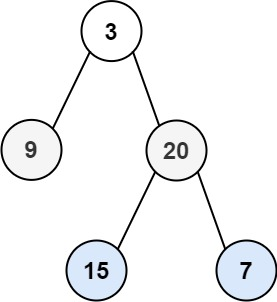So, here we would first print the root (3) and then traverse right to left and print [20,9] and then finally traverse left to right printing [15,7], completing the ZigZag traversal of Binary Tree.

Let's get started and learn various approaches to solve this problem.## Approach - Using Two Stacks

We could achieve ZigZag traversal of Binary Tree by having 2 stacks(Stack - A LIFO data structure) for alternate (left to right) and (right to the left) traversal and then print the nodes alongside.

So,

Step1: We create 2 stacks of type TreeNode. Initially stack1 stores root and stack2 is empty.

We work till both the stacks are not empty.

We then enter inside the loop where we perform the following steps:

• While (Stack1’s size() > 0)
• The popped node’s Right Child (Rc) is entered in stack2 and printed, if it exists.
• The popped node’s Left Child (Lc) is entered in stack2 and printed, if it exists.
• While (Stack2’s size() > 0)
• The popped node’s Left Child (Lc) is entered in stack1 and printed if it exists.
• The popped node’s Right Child (Rc)is entered in stack1 and printed if they exist.

Let us do a dry run on this tree for better understanding: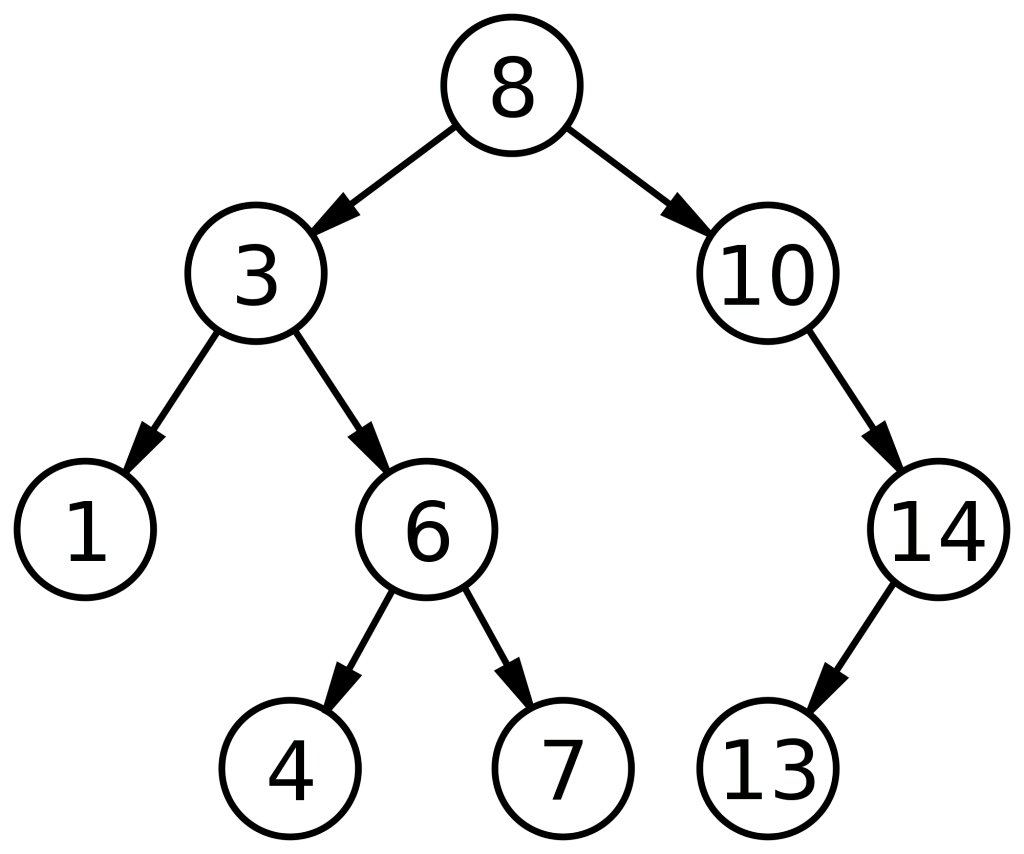Stack1 -  Stack2 - [ ]. ALso,  Root ie 8 is printed.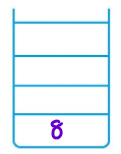We enter the while loop:

1. So,  8 is popped from Stack1 and its Rc(10) and Lc(3) are added in Stack2.

Stack1 =[] Stack2 = [3, 10] and 10 3 are printed.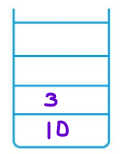2.  So,  3 is popped from Stack2 and its Lc(1) and Rc(6) are added in Stack1.

Stack1 =[6,1] Stack2 =  and 1 6 are printed.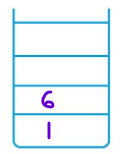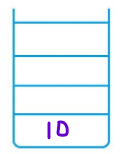3.  10 is popped from Stack2 and its Rc(14) are added in Stack1. (Lc of 10 does not exist).

Stack1 =[14, 6,1] Stack2 = [ ] and 14 is printed.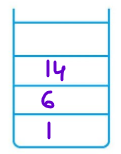4.  Similarly, 14 is popped from Stack1 and its Rc(13) is added in Stack2. (Lc of 14 does not exist).

Stack1 =[6,1] Stack2 =  and 13 is printed.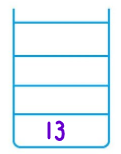5.  6 is popped from Stack1 and its Rc(7) and Lc(4) are added in Stack2.

Stack1 = Stack2 = [4,7,13] and 7, 4 are printed.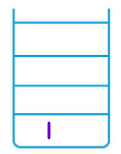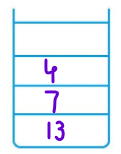6.  1 is popped from Stack1. Lc and Rc of 1 does not exist.

Stack1 =[] Stack2 = [4,7,13]7.  4 is popped from Stack2. Lc and Rc of 4 do not exist.

Stack1 =[] Stack2 = [7,13]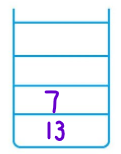8.  7 is popped from Stack1. Lc and Rc of 7 do not exist.

Stack1 =[] Stack2 =9.  13 is popped from Stack1. Lc and Rc of 13 do not exist.

Stack1 =[] Stack2 = []

Since both the stacks are empty, the loop ends.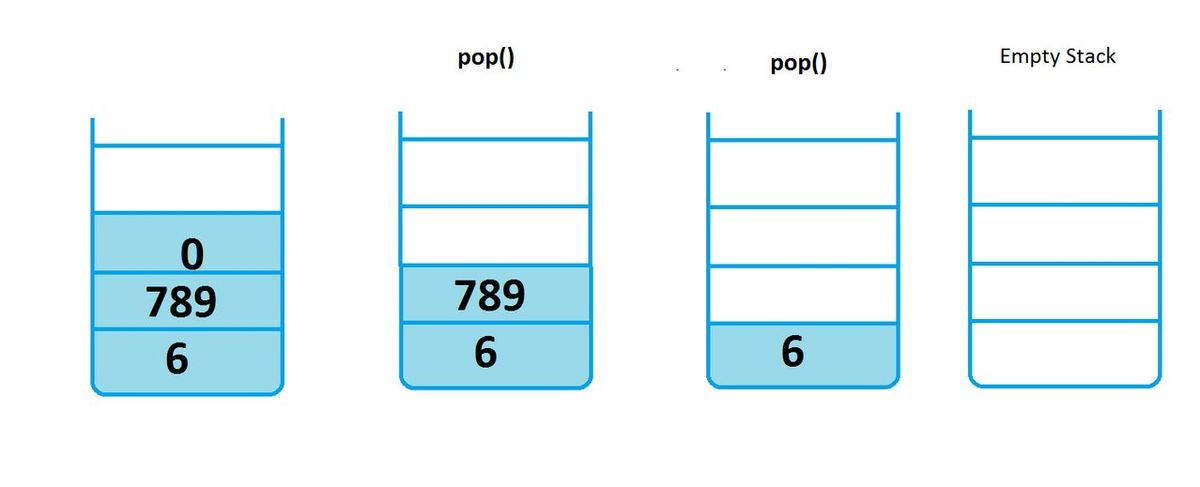Implementation-

Let’s have a look at its implementation in Java -

Output:

## Time and Space Complexity-

Time Complexity: O(n) as we are traversing all the nodes of the Binary tree in ZigZag traversal of Binary Tree at least once.

Space Complexity: O(n) as extra space for storing the nodes of alternate levels in stack has been used.

Where n is the number of nodes in a Binary Tree.

1. What is a Binary Tree?

Ans. A generic tree with at most two child nodes for each parent node is known as a Binary Tree. An empty tree is also considered a valid Binary Tree.

2.  What is the ZigZag traversal of Binary Tree?

Ans.  ZigZag Traversal of a Binary Tree means that we need to print the nodes in the given tree in a zigzag manner, i.e., from left to right, then right to left for next level, and so on, following alternate directions for alternate levels.

3.  What is the best case time complexity for  the ZigZag traversal of Binary Tree?

Ans. The best-case time complexity for ZigZag Traversal of a Binary Tree is O(1), i.e. when only a single or no node is present in the Binary Tree.

4.  What are different possible Traversals for Binary Trees?

Ans: The different possible traversals of Binary Tree are level-order traversal, Diagonal traversal etc.

## Key Takeaways-

In this blog, we learned various approaches for ZigZag traversal of Binary Trees.

• ZigZag Traversal of a Binary Tree means that we need to print the nodes in the given tree in a zigzag manner, i.e., from left to right, then right to left for next level and so on, following alternate directions for alternate levels.
• The minimum space complexity required is O(n) where n = number of nodes in a Binary Tree as we need to store all the nodes of Binary Tree in any used data structure.
• The different possible traversals of Binary Tree are level-order traversal, Diagonal traversal etc.

Check out more blogs on different traversals of Binary Tree like Diagonal Traversal, Level Order Traversal to read more about these topics in detail. And if you liked this blog, share it with your friends!